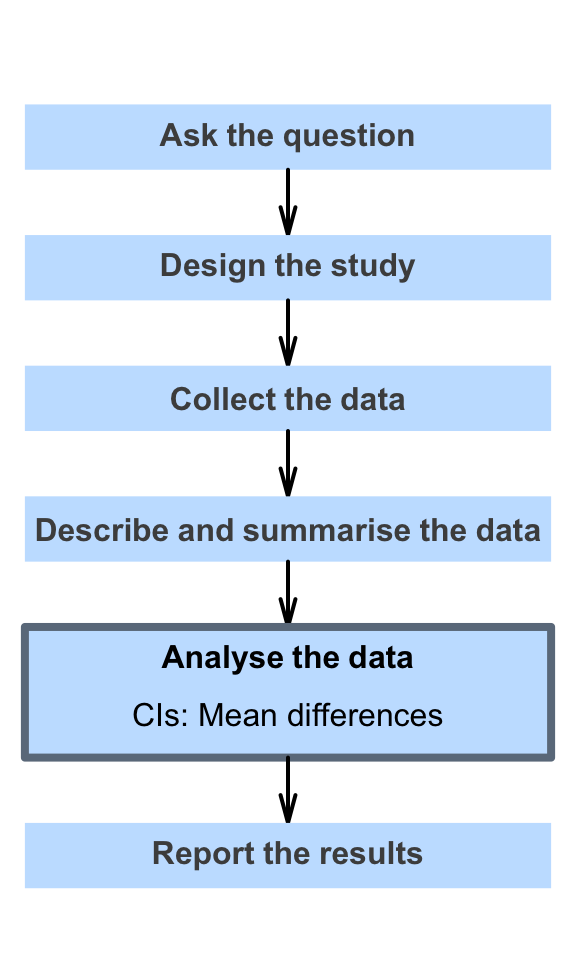# 23 Confidence intervals for mean differences (paired data)

So far, you have learnt to ask a RQ, identify different ways of obtaining data, design the study, collect the data describe the data, summarise data graphically and numerically, and understand the tools of inference.

In this chapter, you will learn about confidence intervals for mean differences (i.e., for paired data). You will learn to:

• produce a confidence interval for a mean difference.
• determine whether the conditions for using the confidence interval apply in a given situation.
• compute sample size estimates in these this situation.